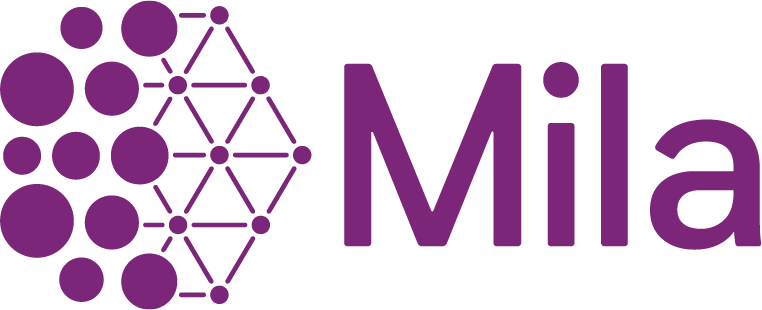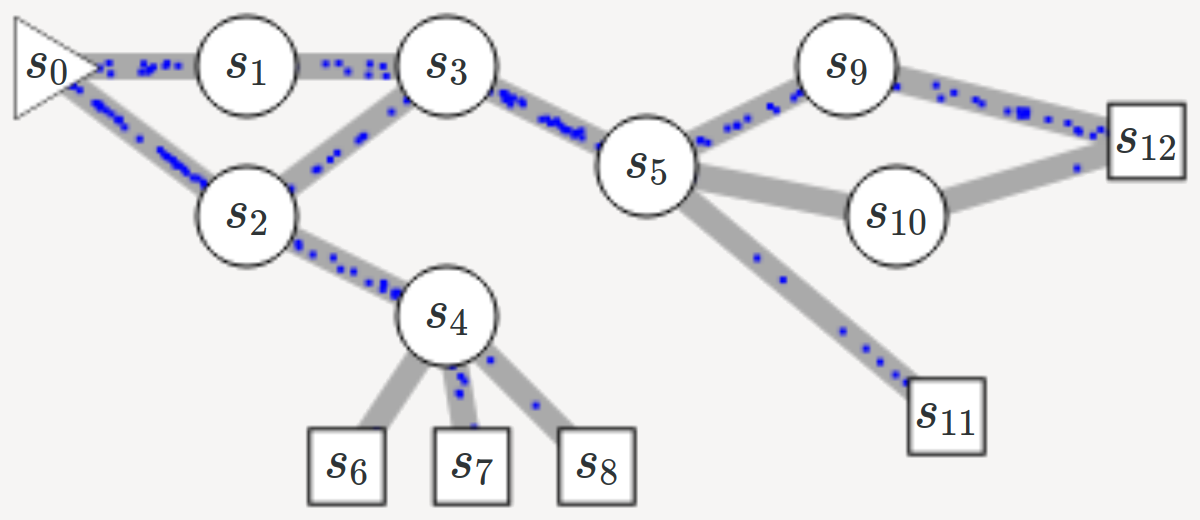name: title class: title, middle

## GFlowNet

### Flow network based generative models for non-iterative diverse candidate generation

Emmanuel Bengio et al., arXiv:2106.04399## Goal

Generate diverse and high-reward samples $x$ from a large search space, given a reward $R(x)$ and a deterministic episodic environment where episodes terminate by generating a sample $x$.

Instead of sampling iteratively, as in Markov chain Monte-Carlo (MCMC methods), Flow Networks sample sequentially, like in episodic Reinforcement Learning (RL), by modelling $\pi(x) \propto R(x)$, that is a distribution proportional to the reward. Unlike RL methods, which generate a single highest-reward sequence, GFlowNet aims to sample multiple, diverse sample.

## Flow Networks

Directed acyclic graph (DAG) with sources and sinks.

.center[]

• A single source node with in-flow $Z$: $s_0$
• Sink nodes are assigned a reward $R(x)$: $s_{6,7,8,11,12}$
• The in-flow of the source node equals the sum of sink node’s out-flow: $\sum_x R(x) = Z$

## Flow Networks

### A generative model

.center[]

Given the structure of the graph and the reward of the sinks, we wish to calculate a_valid flow_ between nodes.

Multiple solutions are possible in general, which yields a generative model

## Flow Networks

### A Markov decision process (MDP)

• States: each node in the graph
• Actions: each edge in the graph

Let

• $Q(s, a) = f(s, s’)$ be the flow between nodes $s$ and $s’$
• $T(s, a) = s’$ be the deterministic state transition to from state $s$ and action $a$.
•  $\pi(a s) = \frac{Q(s,a)}{\sum_{a’} Q(s,a’)}$

Following policy $\pi$ from $s_0$ leads to terminal state $x$ with probability $R(x)$.

## Approximating Flow Networks

### Flow equations

For every node $s’$:

• In-flow: $V(s’) = \sum_{s,a:T(s,a)=s’} Q(s,a)$
• Out-flow: $V(s’) = \sum_{a’ \in \mathcal{A}(s’)} Q(s’,a’)$

The in-flow equals the out-flow, and the out-flow of the sink nodes is their associated reward $R(x) > 0$. Thus:

$\sum^{s,a:T(s,a)=s'} Q(s,a) = R(s')+ \sum^{a' \in \mathcal{A}(s')} Q(s', a')$

A flow that satisfies the flow equations produces $\pi(x) = \frac{R(x)}{Z}$

## Objective function for RL

$\sum^{s,a:T(s,a)=s'} Q(s,a) = R(s')+ \sum^{a' \in \mathcal{A}(s')} Q(s', a')$

The above equation can be seen as a recursive value function. We can approximate the flows such that the conditions are satisfied at convergence (as the Bellman conditions for temporal difference learning):

$\tilde{\mathcal{L}}_{\theta}(\tau) = \sum^{s' \in \tau \neq s_0} \left( \sum^{s,a:T(s,a)=s'} Q(s,a;\theta) - R(s') - \sum^{a' \in \mathcal{A}(s')} Q(s',a';\theta)\right)^2$

For better numerical stability:

$\footnotesize \tilde{\mathcal{L}}_{\theta,\epsilon}(\tau) = \sum^{s' \in \tau \neq s_0} \left( \log \left[ \epsilon + \sum^{s,a:T(s,a)=s'} \exp Q^{log}(s,a;\theta) \right] - \log \left[ \epsilon + R(s') - \sum^{a' \in \mathcal{A}(s')} \exp Q^{log}(s',a';\theta) \right] \right)^2$

## Generating molecules

### Example from the article

Goal: generate a diverse set of small molecules that have high reward.

• Reward: a proxy model that predicts the binding energy of a molecule to a protein target
• States: every possible molecule, made of blocks (SMILES): up to $10^{16}$ states
• Actions: what block to attach to the molecule of a state, and where, plus a stop action: 100-2000 actions